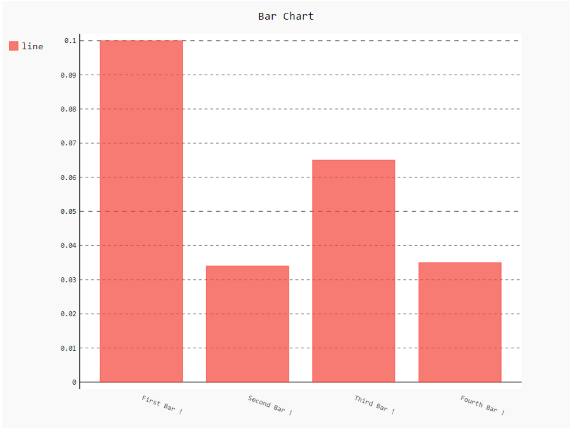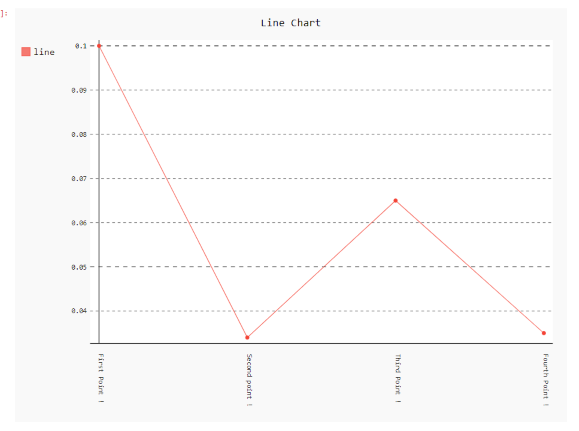GFG App
Open AppBrowser
Continue

# How to rotate x labels using Pygal?

Prerequisites: Pygal

Pygal is a graphics and user interface library for Python that provides functionality commonly required in designing and science applications. While making a plot it is important for us to optimize its label, title, size. In this article, we will see how we can rotate the X-Label value of the plot window in the Pygal module. This can be done by passing the angle of rotation to x_label_rotation parameter.

Here are various ways to change the default plot x_label as per our requirement.

Approach:

• Import required module.
• Create a chart object.
• Pass x_label_rotation value in function.
• Label the graph.
• Display Graph.

Implementation of the concept discussed above is given below:

Example 1:

## Python3

 `# import library ` `import` `pygal ` `import` `numpy ` ` `  `# creating the chart object ` `chart ``=` `pygal.Bar(x_label_rotation``=``20``) ` ` `  `# Random data ` `chart.x_labels ``=` `[ ` `    ``'First Bar !'``, ` `    ``'Second Bar !'``, ` `    ``'Third Bar !'``, ` `    ``'Fourth Bar !'``] ` `chart.add(``'line'``, [``0.1``, .``034``, .``065``, .``035``]) ` ` `  `# naming the title ` `chart.title ``=` `'Bar Chart'` ` `  `chart.render_to_png(``'img.png'``); `

Output:Example 2:

## Python3

 `# importing pygal ` `import` `pygal ` `import` `numpy ` ` `  `# creating the chart object ` `chart ``=` `pygal.Line(x_label_rotation``=``90``) ` ` `  `# Random data ` `chart.x_labels ``=` `[ ` `    ``'First Point !'``, ` `    ``'Second point !'``, ` `    ``'Third Point !'``, ` `    ``'Fourth Point !'``] ` `chart.add(``'line'``, [``0.1``, .``034``, .``065``, .``035``]) ` ` `  `# naming the title ` `chart.title ``=` `'Line Chart'` ` `  `chart.render_to_png(``'img.png'``) `

Output:My Personal Notes arrow_drop_up